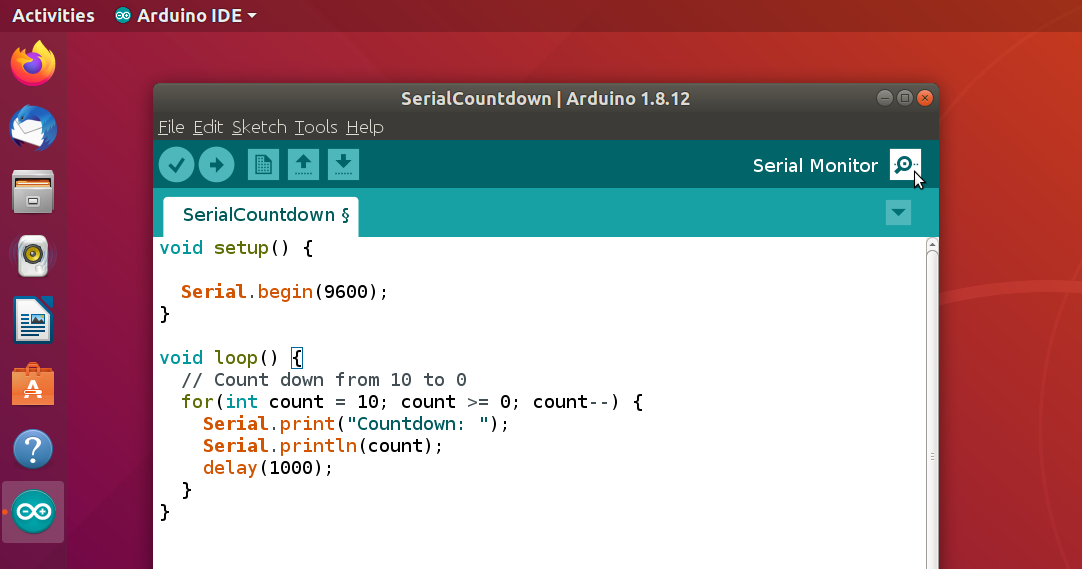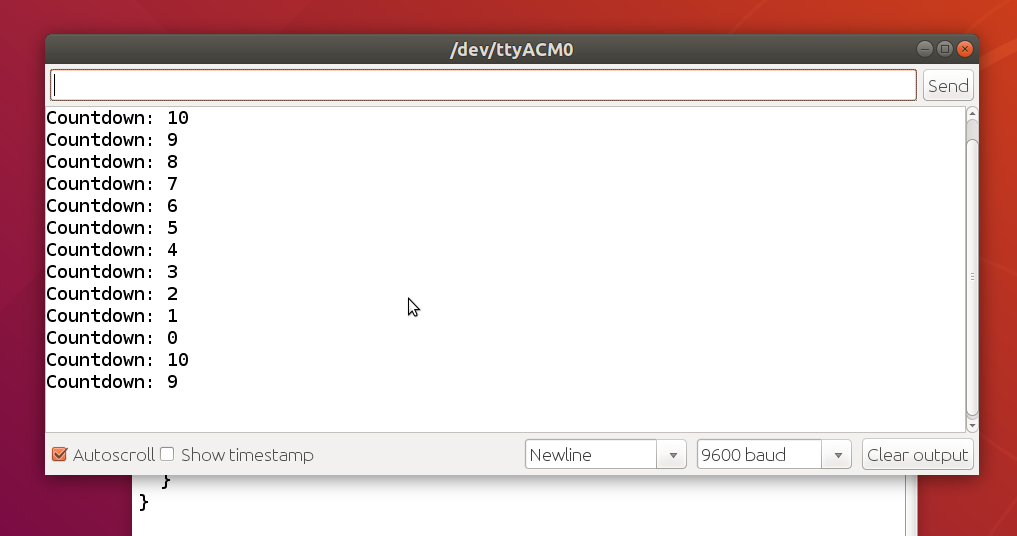# Serial Communication

Let's discover the serial port and learn how to send messages to the computer and receive configuration values.

## What is Serial Communication?

In serial communication bits are transferred one after each other through the wire. This makes it easy to use them with just a few pins. In case of the Universal Serial Asynchronous Transmitter (UART) of the Arduino Uno, there are two pins used for the data transmission itself. These are marked RX (Pin 0) and TX (Pin 1). The communication is bidirectional. The Arduino can receive data through the RX pin and send data through the TX pin, at the same time. In case of the Arduino Uno these pins are also connected to the on-board USB-to-Serial converter, which enables us to directly communicate with the computer.

What are potential use cases for serial communication?
Well, you can use it to transmit measurement data and status messages to the computer. Sending status messages can be very handy when you need to troubleshoot your code. Just send a message with the current values and compare them to your expectations or send a message when a certain point in your code has been reached. You can however, receive data from the computer, as well. This enables us to configure our Arduino programs and even create interactive menus.

Let's keep it simple at this stage. In this tutorial we will create a simple countdown and learn how to configure its start value using our computer. To give you an impression of what can be potentially done, we will turn our Arduino into a small calculator in the end of this tutorial.

## Creating a Countdown

How to create a countdown? Let's divide this problem into two parts: The actual countdown and the serial communication.

For the serial communication, we need to add code to the setup procedure to initialize the communication. For the loop procedure we need code to send the actual messages. Here is a list of procedures we are going to use:

• Serial.begin(baudrate)
• baudrate: Communication speed for the serial port (usually 9600)
• Serial.print(value)
• value: Text, character, integer or floating-point number to send
• Serial.println(value)
• value: Text, character, integer or floating-point number to send

What is the difference between Serial.print and Serial.println? Well, the only difference is that Serial.println causes a line break after the sent value. The Serial.begin function is used to initialize the communication. The speed has to match the speed selected on the computer. Its default setting in the Arduino IDE is 9600 symbols per second. To make it work, we will use the same value in our initialization code.

Now that we now the procedures needed to do serial communication, let's focus on the countdown. We can define a variable, set the initial value e.g. to ten and then use a while loop in which we send the current value and then subtract one from the variable. We would need to this as long as the value is greater or equal zero. But as such an iteration through numbers is a pretty common thing to do, there is also a simpler a cleaner solution for this: The for loop.

for(initialization; condition; loop-statement) {
statement1;
statement2;
}

In the initialization phase of the for loop we can use a single statement to define our counter variable and assign the initial value. The condition determines when the loop ends. Analogous to the while loop, the loop ends as soon as the condition evaluates to false. The loop statement allows us to specify a single statement to change our counter variable after each execution of the loop. For our countdown this will look like this:

for(int count = 10; count >= 0; count--) {
// Do the serial communication here
}

The statement count-- is just a handy shortcut to decrement a value by one. You can do the same thing to increment a variable by one, using count++. There is more than one alternative to reach the same goal: count = count - 1 or count -= 1 will work as well. These two options work for other math operations like division or multiplication, also.

Combined we get the following code for our countdown:

void setup() {
Serial.begin(9600);
}

void loop() {
// Count down from 10 to 0
for(int count = 10; count >= 0; count--) {
Serial.print("Countdown: ");
Serial.println(count);
delay(1000);
}
}

Let's look at our result. To do so, we need to open the serial monitor of the Arduino IDE. Click the button in the right corner of the header bar, as shown in the following screenshot:In the serial monitor we can watch the message sent by the Arduino Uno. We can use the text field at the top to write a message and send it. We will need this for the next steps.## Restart on Command

A bit annoying in our current solution is the fact that the countdown starts again after reaching zero. How can we fix it? The simplest thing would be to add a loop that runs forever to the end the program:

while(true);

This way the countdown will not restart except when we reset the Arduino Uno. But that would be pretty boring, wouldn't it? Since we can also send data to the Arduino, why not let it wait for a key press to restart? To do so we can use the following command:

• Serial.available()
• Returns true when data was received from the computer

We can add a loop, waiting for this function to return true, to the end of out program code. When you click 'Send' in the serial monitor the countdown will restart. There will be the following problem, however: After you send something once, the countdown will not stop anymore. This is because the data send in the past is still there. We need to read them to empty the input buffer. We can use the Serial.read function for this:

• Serial.read()
• Returns the character sent or -1 if no more data is available

To clear the buffer we just loop until -1 is returned. The adjusted code looks like this:

void setup() {
Serial.begin(9600);
}

void loop() {
// Count down from 10 to 0
for(int count = 10; count >= 0; count--) {
Serial.print("Countdown: ");
Serial.println(count);
delay(1000);
}

while(Serial.available() == 0);
}

## Configure it

Why just read and drop the received bytes? We can also use the input from the computer to configure at which value the countdown starts. For this use case the following function will come in handy:

• Serial.parseInt()
• Reads and returns an integer of type long

Note that we can assign different integer types as well as floats and integers to each other directly. The type will be implicitly converted and the value is truncated or will overflow, depending on what you like to call it, if it doesn't fit. In the following code sample I used the type int even though the Serial.parseInt function will return an integer with type long. By using this function we can read the start value at the beginning. We still need to drop the remaining bytes, as there will be a newline character send just after the number. I save the value in a variable which is later used to set the initial counter value.

void setup() {
Serial.begin(9600);
}

void loop() {
while(Serial.available() == 0);
int startValue = Serial.parseInt();

// Count down from startValue to 0
for(int count = startValue; count >= 0; count--) {
Serial.print("Countdown: ");
Serial.println(count);
delay(1000);
}
}

## A Simple Calculator

Last but not least, the promised example code for a simple calculator:

void setup() {
Serial.begin(9600);
}

void loop() {
while(Serial.available() == 0);

int n1 = Serial.parseInt();
int n2 = Serial.parseInt();

if(op == '+') {
Serial.println(n1+n2);
}
else if(op == '-') {
Serial.println(n1-n2);
}
else if(op == '*') {
Serial.println(n1*n2);
}
else if(op == '/') {
Serial.println(n1/n2);
}
else {
Serial.println("Invalid input, try again!");
}
}

With the knowledge you gained throughout this tutorial, it should not be hard to understand how it works. The code waits for user input and then reads an integer followed by the operation and a second number. The if-else construction is used to determine the chosen operation for which the result is calculated and send back to the user.

To use it, just enter your calculation task (e.g. 1+1) in the text field of the serial monitor and send it to the Arduino. The Arduino will respond with the result of your calculation. Note that you will get incorrect results when using larger numbers. This is due to the integer overflow I spoke about in the last tutorial. To allow larger numbers replace the type int with long.